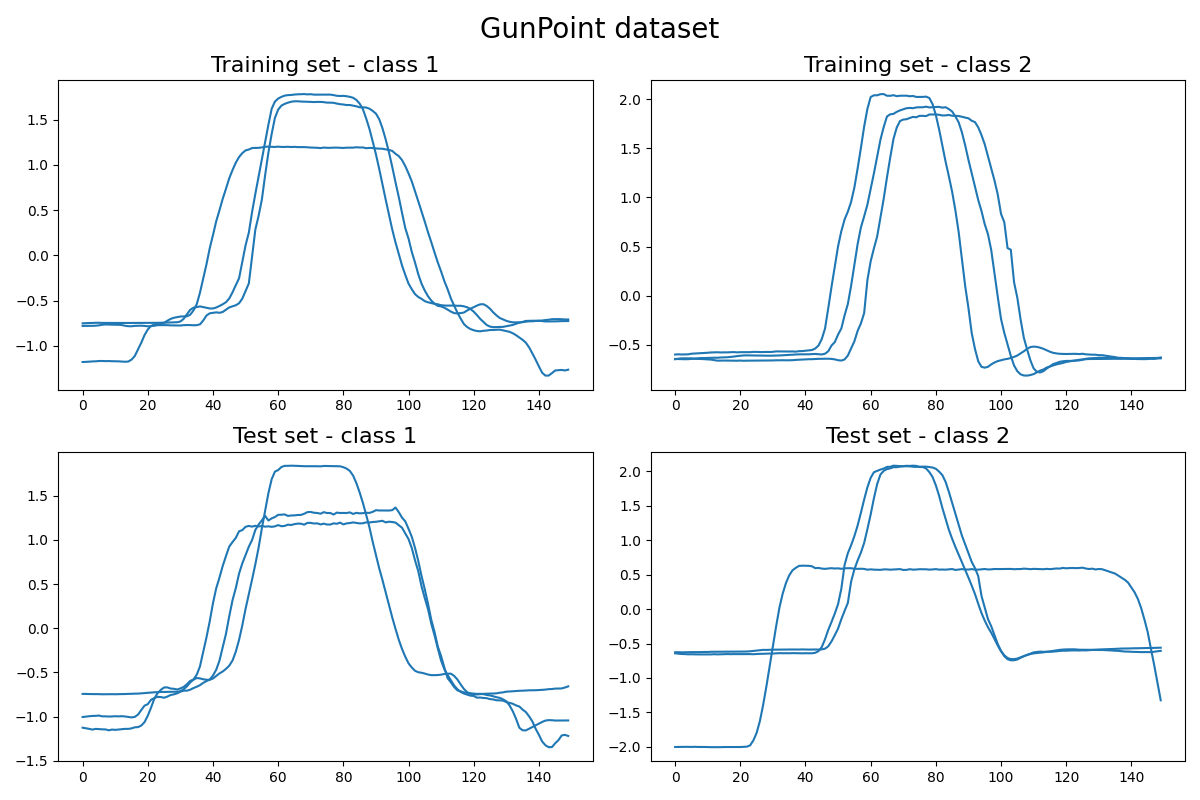This example shows how to load and plot the GunPoint dataset. This dataset involves one female actor and one male actor making a motion with their hand. The two classes are: Gun-Draw and Point: For Gun-Draw the actors have their hands by their sides. They draw a replicate gun from a hip-mounted holster, point it at a target for approximately one second, then return the gun to the holster, and their hands to their sides. For Point the actors have their gun by their sides. They point with their index fingers to a target for approximately one second, and then return their hands to their sides. For both classes, we tracked the centroid of the actor’s right hands in both X- and Y-axes, which appear to be highly correlated. The data in the archive is just the X-axis. It is implemented as `pyts.datasets.load_gunpoint()`.```# Author: Johann Faouzi <johann.faouzi@gmail.com>

import matplotlib.pyplot as plt

X_train, X_test, y_train, y_test = load_gunpoint(return_X_y=True)
n_samples_per_plot = 3

plt.figure(figsize=(12, 8))

for i, (X, y, set_, class_,) in enumerate(zip(
[X_train, X_train, X_test, X_test],
[y_train, y_train, y_test, y_test],
['Training', 'Training', 'Test', 'Test'],
[1, 2, 1, 2]
)):
plt.subplot(2, 2, i + 1)
for j in range(n_samples_per_plot):
plt.plot(X[y == class_][j], 'C0')
plt.title('{} set - class {}'.format(set_, class_), fontsize=16)

plt.suptitle('GunPoint dataset', fontsize=20)
plt.tight_layout()